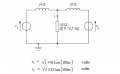# Trouble calculating voltage

#### Fnordgasm5

Joined Mar 2, 2013
5Above is the circuit from one of my assignments. I am asked to calculate the current through the load first using superposition and again using Thevenin's theorem.

Using superposition is not a problem. My issue is calculating the equivelent Thevenin's voltage for the circuit. Specifically I am having a hard time conceptualising what is happening at the dot between the two inductors when the load is removed. Is the voltage the sum of the two voltages entering the node or am I subtracting the two? I seem to have arrived at a value of 586.9V at an angle of 135 degrees but for the love of god I could not tell you how I managed that.

#### Jony130

Joined Feb 17, 2009
5,155
What if you replace the inductors in a circuit and use resistors instead. Are you now able to solve for the voltage at the "middle" point?